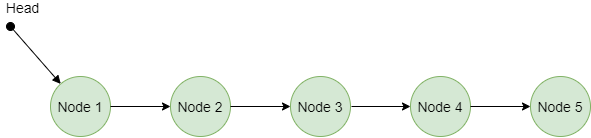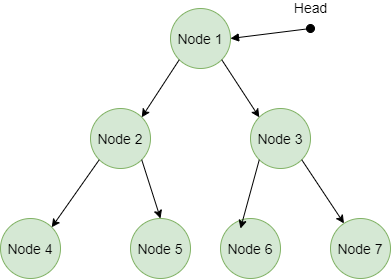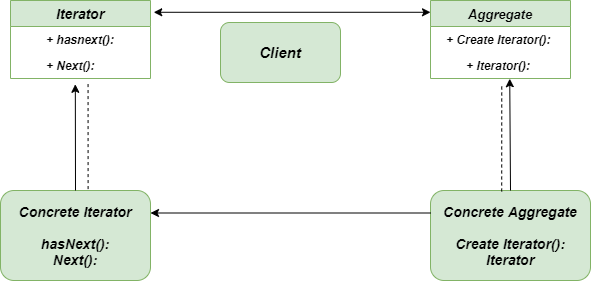Open In App
Related Articles

# Iterator Method – Python Design Patterns

Iterator method is a Behavioral Design Pattern that allows us to traverse the elements of the collections without taking the exposure of in-depth details of the elements. It provides a way to access the elements of complex data structure sequentially without repeating them.
According to GangOfFour, Iterator Pattern is used ” to access the elements of an aggregate object sequentially without exposing its underlying implementation”.

The following diagram depicts the Linked List data structure.### Problem Without using Iterator Method

Imagine you are creating an application for small kids which takes any valid alphabet as input and return all the alphabets up to that. When this application will be used only a few times, it is okay to run For loop and While loop again and again but when the frequency of running increases this process becomes quite inefficient. So, we have to find a way in order to avoid these loops. This problem may become bigger when we will work on complex non-linear data structures like Trees, Graphs where traversing is not that simple as in an array.
The following diagram depicts the image of the Tree data structure.Iterator-Method-Tree-Data-Structure

### Solution Using Iterator Method

Here we will discuss the solution for the above-described problem. It’s always handy for Python users to use Iterators for traversing any kind of data structure doesn’t matter they are linear or no-linear data structures. We have two options to implement Iterators in Python either we can use the in-built iterators to produce the fruitful output or explicitly we can create iterators with the help of Generators. In the following code, we have explicitly created the Iterators with the help of generators.

Note: Following code is the example of an explicitly created Iterator method

## Python3

 `""" helper method for iterator"""`  `def` `alphabets_upto(letter):``    ``"""Counts by word numbers, up to a maximum of five"""``    ``for` `i ``in` `range``(``65``, ``ord``(letter)``+``1``):``            ``yield` `chr``(i)`  `"""main method"""``if` `__name__ ``=``=` `"__main__"``:` `    ``alphabets_upto_K ``=` `alphabets_upto(``'K'``)``    ``alphabets_upto_M ``=` `alphabets_upto(``'M'``)` `    ``for` `alpha ``in` `alphabets_upto_K:``        ``print``(alpha, end``=``" "``)` `    ``print``()` `    ``for` `alpha ``in` `alphabets_upto_M:``        ``print``(alpha, end``=``" "``)`

Note: Following code is the example of using an in-built iterator method

## Python3

 `"""utility function"""``def` `inBuilt_Iterator1():``    ` `    ``alphabets ``=` `[``chr``(i) ``for` `i ``in` `range``(``65``, ``91``)]``    ` `    ``"""using in-built iterator"""``    ``for` `alpha ``in` `alphabets:``        ``print``(alpha, end ``=` `" "``)``    ``print``()` `"""utility function"""``def` `inBuilt_Iterator2():``    ` `    ``alphabets ``=` `[``chr``(i) ``for` `i ``in` `range``(``97``, ``123``)]``    ` `    ``"""using in-built iterator"""``    ``for` `alpha ``in` `alphabets:``        ``print``(alpha, end ``=` `" "``)``    ``print``()`  `"""main method"""``if` `__name__ ``=``=` `"__main__"` `:``    ` `    ``"""call the inbuiltIterators"""``    ``inBuilt_Iterator1()``    ``inBuilt_Iterator2()`

### Class Diagram

Following is the class diagram for the Iterator MethodIterator-Method-Class-Diagram

• Single Responsibility Principle: It’s really easy to extract the huge algorithms into separate classes in the Iterator method.
• Open/Closed Principle: Passing the new iterators and collections into the client code does not break the code can easily be installed into it.
• Easy to use Interface: It makes the interface really simple to use and also supports the variations in the traversal of the collections.

• Unnecessary Wasting resources: It’s not always a good habit to use the Iterator Method because sometimes it may prove as an overkill of resources in a simple application where complex things are not required.
• Increases Complexity: As we said earlier, using the Iterator method makes simple applications complex.
• Decreases Efficiency: Accessing elements directly is a much better option as compared to accessing elements using the iterator in terms of efficiency.

### Applicability

• Limited Exposure: When you want to access the elements at the lower level i.e., you are not interested in the internal implementation of the elements then it’s always preferred to use the Iterator Method.
• Traversing Unknown Data Structures: The iterator method can be easily used to traverse various types of data structures such as Trees, Graphs, Stack, Queue, etc. as the code provides a couple of generic interfaces for both collections and iterators.

Further Read – Iterator Method in Java